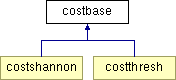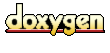Main Page   Class Hierarchy   Compound List   File List   Compound Members   File Members

# costbase Class Reference

Base class for objects that define costs functions for the wavelet packet transform. More...

`#include <costbase.h>`

Inheritance diagram for costbase::List of all members.

## Public Methods

costbase ()
The default constructor does nothing. More...

## Protected Methods

void traverse (packnode< double > *node)
Recursively traverse the wavelet packet tree and calculate the cost function. More...

virtual double costCalc (packnode< double > *node)=0
Cost function to be defined by the subclass. More...

## Private Methods

costbase (const costbase &rhs)
disallow the copy constructor. More...

## Detailed Description

Base class for objects that define costs functions for the wavelet packet transform.

The costbase base class provides a constructor that is passed the root of a wavelet packet tree (which is built by packtree.cpp). The wavelet packet tree is constructed from packnode objects (which are a subclass of the packdata).

The cost function calculation invoked by the constructor traverses the wavelet packet tree (top down) and calls costCalc on each node in the tree. The cost function result is stored in the node. Note that the cost function also calculates a cost value for the original data, since in theory the original data may represent the minimal representation for the data in terms of the cost function.

The pure virtual function costCalc, which calculates the cost function, must be defined by the subclass.

A description of the cost functions associated with the wavelet packet transform can be found in Chapter 8 of Ripples in Mathematics by Jense and la Cour-Harbo.

Author:
Ian Kaplan

Definition at line 68 of file costbase.h.

## Constructor & Destructor Documentation

 costbase::costbase ( const costbase & rhs )` [inline, private]`
 disallow the copy constructor. Definition at line 72 of file costbase.h.```00072 {} ```

 costbase::costbase ( )` [inline]`
 The default constructor does nothing. Definition at line 95 of file costbase.h.```00095 {} ```

## Member Function Documentation

 double costbase::costCalc ( packnode< double > * node )` [protected, pure virtual]`
 Cost function to be defined by the subclass. Reimplemented in costshannon, and costthresh. Referenced by traverse().

 void costbase::traverse ( packnode< double > * node )` [inline, protected]`
 Recursively traverse the wavelet packet tree and calculate the cost function. Definition at line 79 of file costbase.h. Referenced by costshannon::costshannon(), and costthresh::costthresh(). ```00080 { 00081 if (node != 0) { 00082 double cost = costCalc( node ); 00083 node->cost( cost ); 00084 00085 traverse( node->lhsChild() ); 00086 traverse( node->rhsChild() ); 00087 } 00088 } // traverse ```

The documentation for this class was generated from the following file:
Generated at Sat Aug 10 13:23:36 2002 for Wavelet Packet Transform and Lossless Compression by1.2.8.1 written by Dimitri van Heesch, © 1997-2001